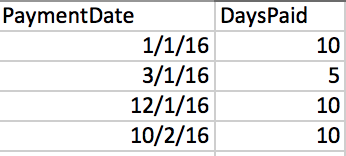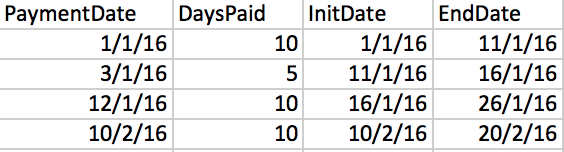# How to calculate values by row, using calculated (so not fixed) values from the previous row

I need to calculate values by rows and after a row is updated, to update the next row using data calculated on the previous row

Let me explain with a simple example :

1.- Imagine a source with two columns, one about the payment date and another with the number of days you buy … something like this :2.- I would like to calculate the period of time this particular customer has been paid, taking into account that the payment can be produced days before the previous period finished.
On an spreadsheet that’s easy to do it, since the formulas are calculated by rows and not by columns :

InitDate = max(EndDate_previous_row,PaymentDate)
EndDate = InitDate + DaysPaidHow can I implement a similar behavior with exploratory ?
I’ve tried to do something similar, using the lag() function to get a value from the previous row, but, since all the values of a column are calculated before the values of the row that has to be used on the formula, the result is not the expected one :

Looks those two calculated columns are cross referencing so it won’t work.

InitDate needs EndDate but EndDate needs InitDate, so that’s logically impossible.

Could be a cross referencing … and maybe that’s the reason because I don’t find an easy way to solve it.

On an spreadsheet I can calculate it easily (so it’s not logically impossible), since the formulas are executed by rows instead than be executed doing column transformations.

The EndDate of the first row is calculated before the InitDate of the second row, and then the EndDate of the second row, and so on …

It’s like to do a cumsum with dates and using two columns … although I didn’t find the way to do it … yet.

You can do something like this.

1. Calculate EndDate first.

EndDate = PaymentDate + DaysPaid

1. Get the previous EndDate by using ‘lag’ function.

EndDate_previous = coalesce(lag(EndDate), EndDate)

I’m using ‘coalesce’ function in case there is no value, that is for the 1st row.

1. Use ‘if_else’ function to compare PaymenDate and EndDate_previous and return the bigger value.

InitDate = if_else(PaymentDate >= EndDate_previous, PaymentDate, EndDate_previous))

Those left hand side of the equal signs are the column name. When you do in Exploratory UI, you don’t want to copy the whole thing, you want to use only the right hand side in the calculation input area.

Let me know if this works.